# FORM ONE MATHEMATICS STUDY NOTES TOPIC 7-9.

0
328TOPIC 7: ALGEBRA

An
algebraic expression – is a collection of numbers, variables, operators
and grouping symbols.Variables – are letters used to represent one or
more numbers
Symbols to form Algebraic Expressions
Use symbols to form algebraic expressions
The parts of an expression collected together are called terms
Example
• x + 2x – are called like terms because they have the same variables
• 5x +9y – are called unlike terms because they have different variables
An algebraic expression can be evaluated by replacing or substituting the numbers in the variables
Example 1
Evaluate the expressions below, given that x = 2 and y = 3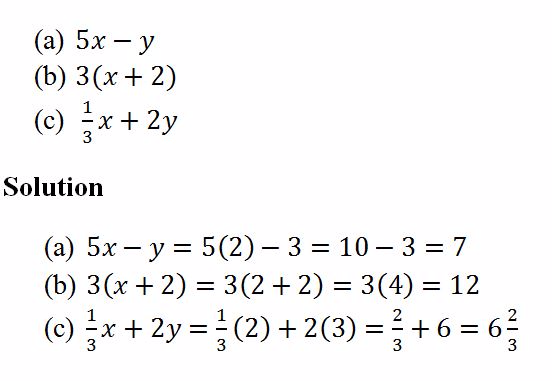Example 2
Evaluate the expressions below, given that m = 1 and n = – 2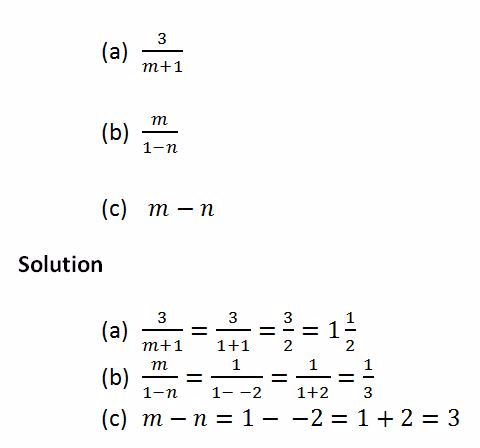An expression can also be made from word problems by using letters and numbers
Example 3
A rectangle is 5 cm long and w cm wide. What is its area?
Solution
Let the area be A.
Then
A = length× widith
A = 5w cm2
Simplifying Algebraic Expressions
Simplify algebraic expressions
Algebraic expressions can be simplified by addition, subtraction, multiplication and division
Addition and subtraction of algebraic expression is done by adding or subtracting the coefficients of the like terms or letters
Coefficient of the letter – is the number multiplying the letter
<!–[endif]–>Multiplication and division of algebraic expression is done on the coefficients of both like and unlike terms or letters
Example 4
Simplify the expressions below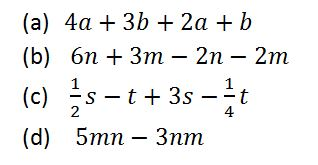Solution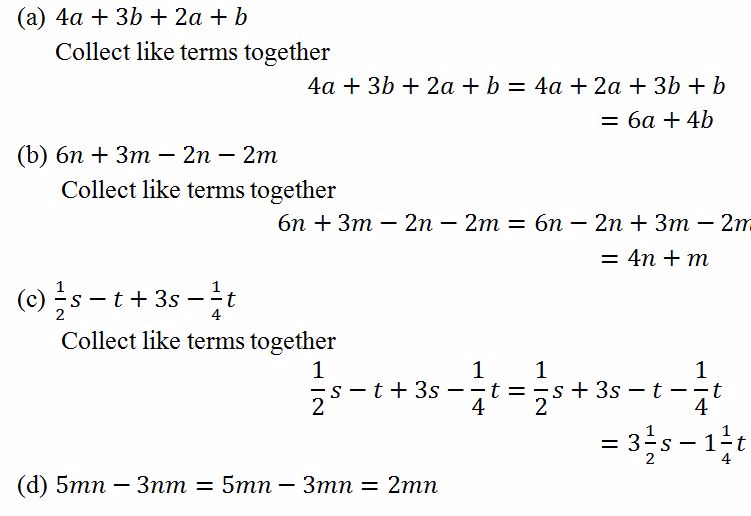An
inequality – is a mathematical statement containing two expressions
which are not equal. One expression may be less or greater than the
other.The expressions are connected by the inequality symbols<,>,≤
or≥.Where< = less than,> = greater than,≤ = less or equal and ≥ =
greater or equal.
Linear Inequalities with One Unknown
Solve linear inequalities in one unknown
An
inequality can be solved by collecting like terms on one side.Addition
and subtraction of the terms in the inequality does not change the
direction of the inequality.Multiplication and division of the sides of
the inequality by a positive number does not change the direction of the
inequality.But multiplication and division of the sides of the
inequality by a negative number changes the direction of the inequality
Example 11
Solve the following inequalities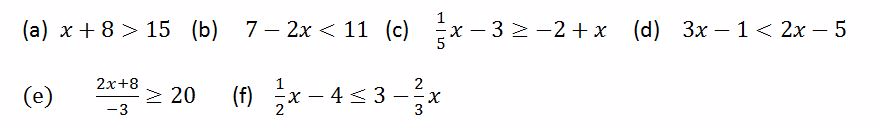Solution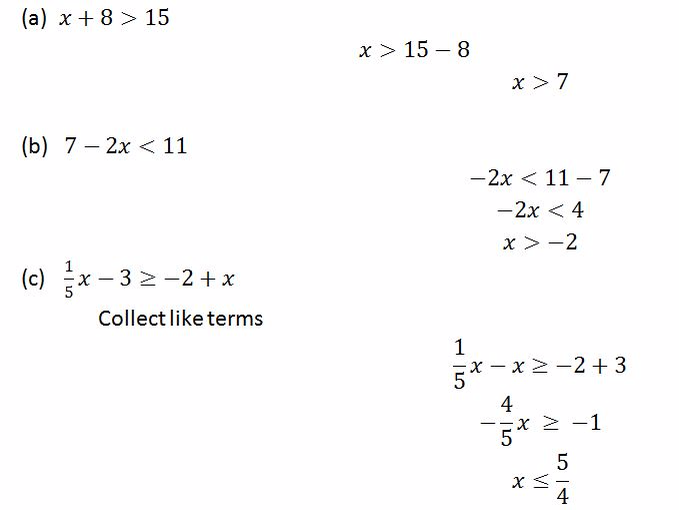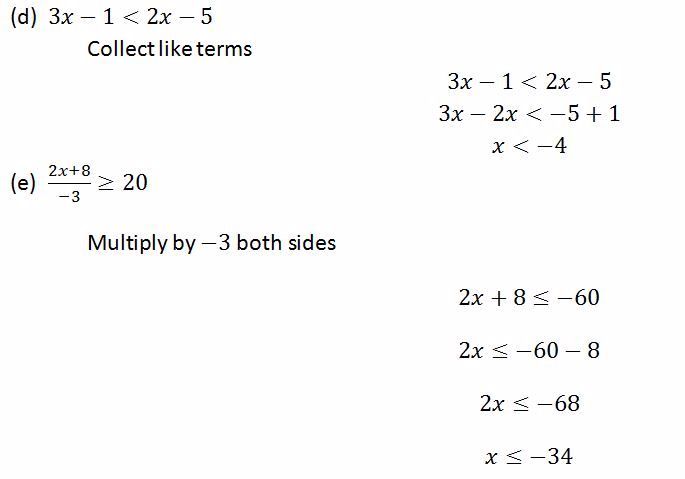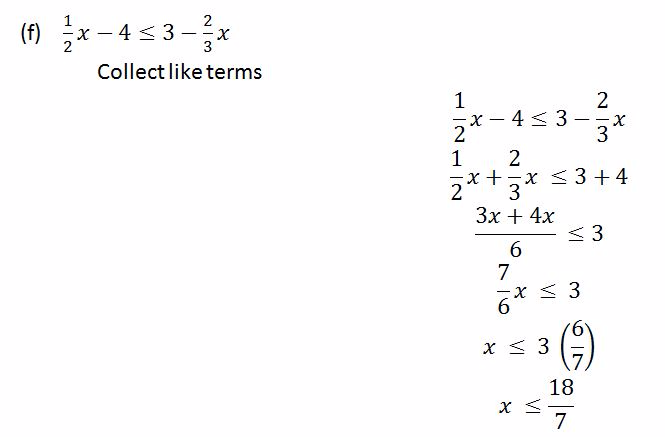Linear Inequalities from Practical Situations
Form linear inequalities from practical situations
To represent an inequality on a number line, the following are important to be considered:
• The endpoint which is not included is marked with an empty circle
• The endpoint which is included is marked with a solid circle
Example 12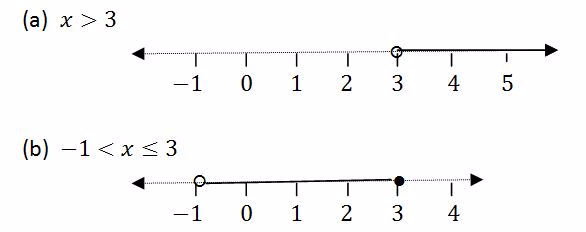Compound statement – is a statement made up of two or more inequalities
Example 13
Solve the following compound inequalities and represent the answer on the number line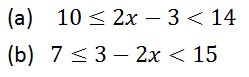Solution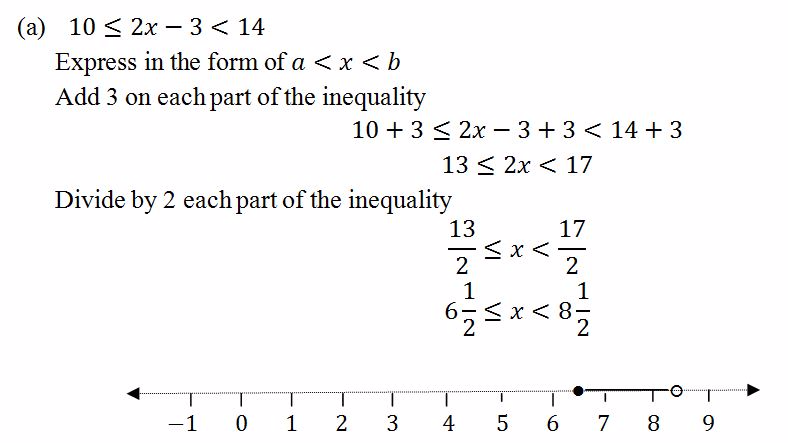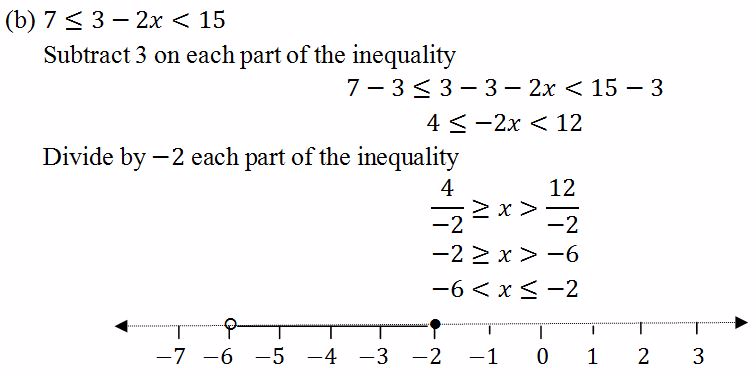TOPIC 8: NUMBERS

A Rational Number
Define a rational number
ARational Numberis a real number that can be written as a simple fraction (i.e. as aratio). Most numbers we use in everyday life are Rational Numbers.
Number As a Fraction Rational?
5 5/1 Yes
1.75 7/4 Yes
.001 1/1000 Yes
-0.1 -1/10 Yes
0.111… 1/9 Yes
√2(square root of 2) ? NO !
The square root of 2 cannot be written as a simple fraction! And there are many more such numbers, and because they arenot rationalthey are calledIrrational.
The Basic Operations on Rational Numbers
Perform the basic operations on rational numbers
To
add two or morerational numbers, the denominator of all the rational
numbers should be the same. If the denominators of all rational numbers
are same, then you can simply add all the numerators and the denominator
value will the same. If all the denominator values are not the same,
then you have to make the denominator value as same, by multiplying the
numerator and denominator value by a common factor.
Example 1
1⁄3+4⁄3=5⁄3
1⁄3 +1⁄5=5⁄15 +3⁄15 =8⁄15
Subtraction of Rational Numbers:
To
subtract two or more rational numbers, the denominator of all the
rational numbers should be the same. If the denominators of all rational
numbers are same, then you can simply subtract the numerators and the
denominator value will the same. If all the denominator values are not
the same, then you have to make the denominator value as same by
multiplying the numerator and denominator value by a common factor.
Example 2
4⁄3 -2⁄3 =2⁄3
1⁄3-1⁄5=5⁄15-3⁄15=2⁄15
Multiplication of Rational Numbers:
Multiplication
of rational numbers is very easy. You should simply multiply all the
numerators and it will be the resulting numerator and multiply all the
denominators and it will be the resulting denominator.
Example 3
4⁄3×2⁄3=8⁄9
Division of Rational Numbers:
Division
of rational numbers requires multiplication of rational numbers. If you
are dividing two rational numbers, then take the reciprocal of the
second rational number and multiply it with the first rational number.
Example 4
4⁄3÷2⁄5=4⁄3×5⁄2=20⁄6=10⁄3